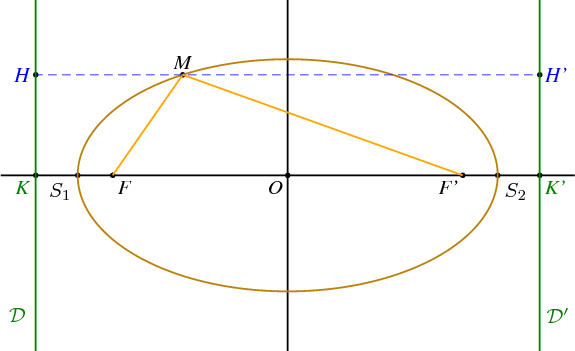Fichier 012.jps — Modifié le 11 Mars 2006 à 10 h 52Source
%% PhS
%%

50 setxunit
-5 5  setxrange
-2.5 2.5 setyrange

%%%%%%%%%%%%%%%%%%%%%%%%%%%%%%%%%%%%%%%%%%%%%%%
%% procédures
%%%%%%%%%%%%%%%%%%%%%%%%%%%%%%%%%%%%%%%%%%%%%%%

/bddroite {
8 dict begin
/kd exch def
/kg exch def
/By exch def
/Bx exch def
/B {Bx By} def
/Ay exch def
/Ax exch def
/A {Ax Ay} def
gsave
[A A B vecteur kg mulv addv A A B vecteur kd mulv addv] ligne
grestore
end
} def

%%%%%%%%%%%%%%%%%%%%%%%%%%%%%%%%%%%%%%%%%%%%%%%
%% definition d'une couleur
%%%%%%%%%%%%%%%%%%%%%%%%%%%%%%%%%%%%%%%%%%%%%%%

/coul1 {120 255 div 70 255 div 9 255 div setrgbcolor} def

%%%%%%%%%%%%%%%%%%%%%%%%%%%%%%%%%%%%%%%%%%%%%%%
%% definition des points
%%%%%%%%%%%%%%%%%%%%%%%%%%%%%%%%%%%%%%%%%%%%%%%

/a {3} def
/c {2.5} def
/b {a a mul c c mul sub sqrt} def
/e {c a div} def
/Kx {a e div} def
/K {Kx neg 0} def
/K+ {K 0 6 addv} def
/K- {K 0 -6 addv} def
/Kp {Kx 0} def
/Kp+ {Kp 0 6 addv} def
/Kp- {Kp 0 -6 addv} def
/O {0 0} def
/F {c neg 0} def
/Fp {c 0} def
/S1 {a neg 0} def
/S2 {a 0} def
/B {0 b} def

/xdet {settvar t cos a mul} def
/ydet {settvar t sin b mul} def

/Mx {120 xdet} def
/My {120 ydet} def
/M {Mx My} def
/H {M K K+ orthoproj} def
/Hp {M Kp Kp+ orthoproj} def

/bissec {F M Fp bissectrice} def
/bixx {bissec O S2 interdroite}def
/Mbixx {M bixx vecteur} def
/T {bissec M perp  Kp Kp+ interdroite} def
/MT {M T vecteur} def

/theta1 {c neg a div arccos} def
/Fcons1 {theta1 xdet theta1 ydet} def
/Fcons2 {theta1 neg xdet theta1 neg ydet} def

%%%%%%%%%%%%%%%%%%%%%%%%%%%%%%%%%%%%%%%%%%%%%%%
%% traces
%%%%%%%%%%%%%%%%%%%%%%%%%%%%%%%%%%%%%%%%%%%%%%%
/coul1 {188 255 div 128 255 div 9 255 div setrgbcolor} def

1.2 setlinewidth
[O F Fp S1 S2 K Kp M H Hp] points

coul1
0 360 settrange
{xdet} {ydet} courbeparam

vert
[K+ K-] ligne
[Kp+ Kp-] ligne

orange
[F M Fp] ligne

0.6 setlinewidth
bleu
pointilles
[H Hp] ligne

%%%%%%%%%%%%%%%%%%%%%%%%%%%%%%%%%%%%%%%%%%%%%%%
%% texte
%%%%%%%%%%%%%%%%%%%%%%%%%%%%%%%%%%%%%%%%%%%%%%%

setTimesItalic
noir
(O) O [1.5 dup] dltext
(F) F [1.5 dup] drtext
(F') Fp [1.5 dup] dltext
(M) M [1.5 dup] uctext
bleu
(H) H [1.5 dup] cltext
(H') Hp [1.5 dup] crtext

noir
<latex>
$S_1$
</latex>
S1 [1.5 dup] dltexlabel

<latex>
$S_2$
</latex>
S2 [1.5 dup] drtexlabel

vert
(K) K [1.5 dup] dltext
(K') Kp [1.5 dup] drtext

<latex>
$\mathcal{D}$
</latex>
(D) K 0 -2 addv [1.5 dup] cltexlabel

<latex>
$\mathcal{D'}$
</latex>
(D) Kp 0 -2 addv [1.5 dup] crtexlabel